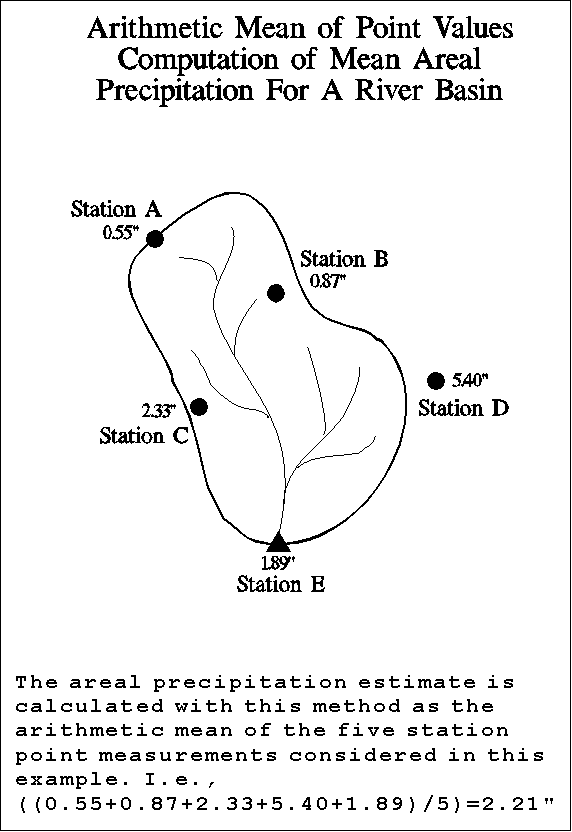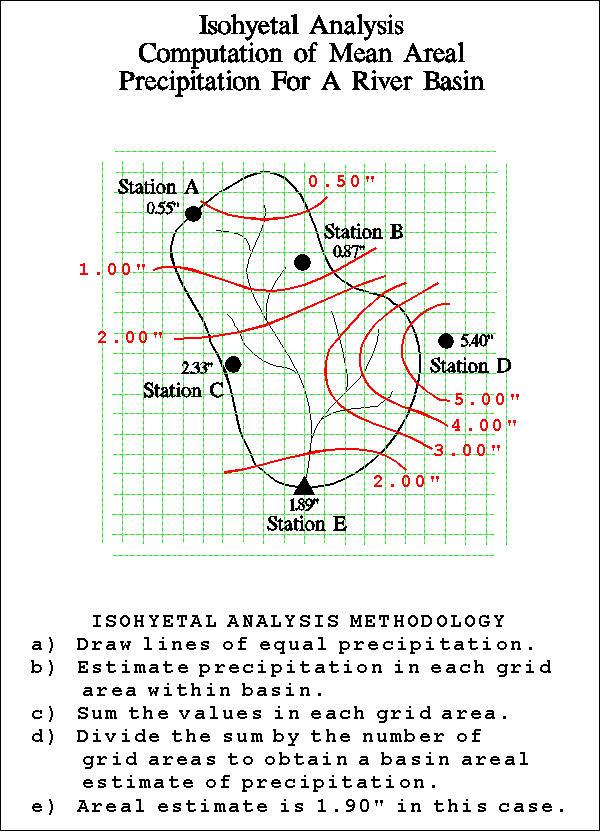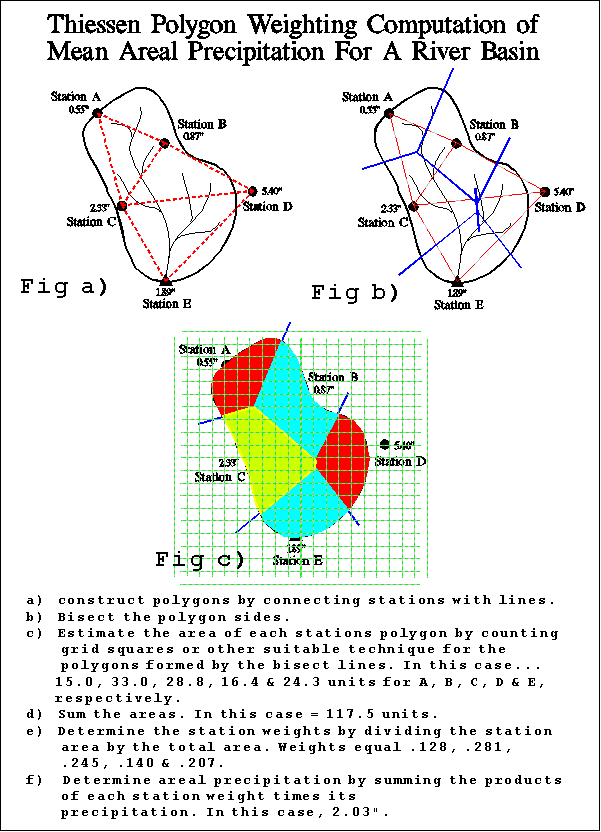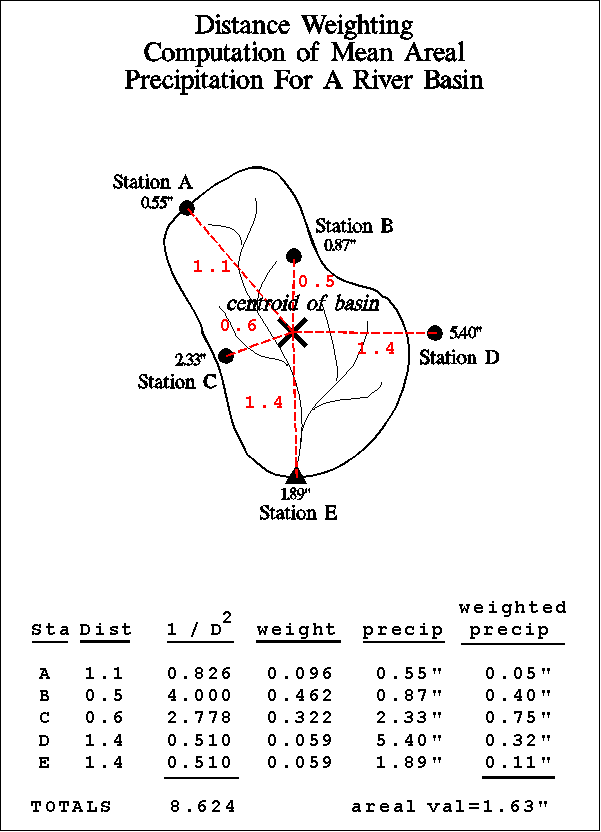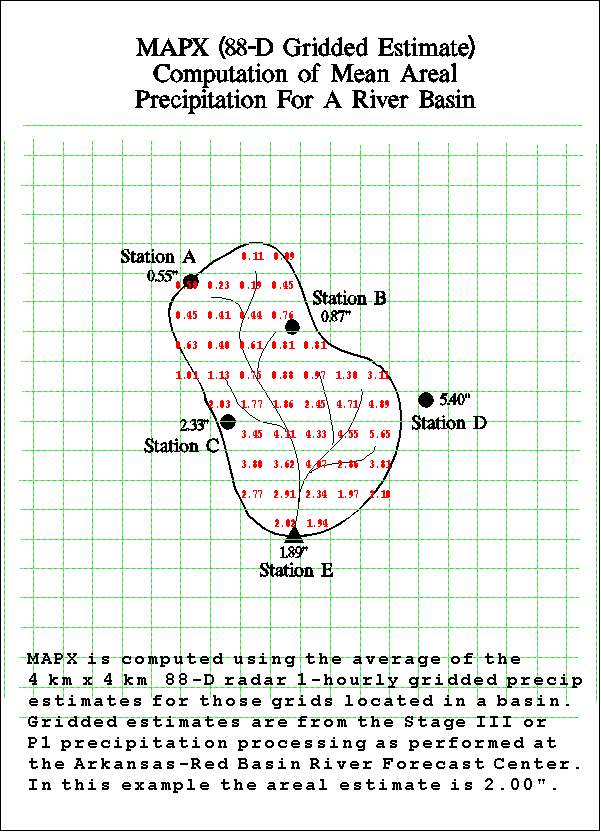## Point Precipitation Measurement,  Areal Precipitation Estimates And  Relationships To Hydrologic Modeling

Precipitation Measurement
Liquid precipitation is traditionally measured using various types of rain gages such as the non-recording cylindrical container type or the recording weighing type, float type and tipping-bucket type. All of the above gages measure precipitation at a point. Another method of measuring precipitation is through the use of radar. The actual measurement taken by the radar is of backscattered power of the echo returns. This return power is used to calculate a reflectivity factor, Z. The Z reflectivity factor is then used in an equation referred to as a "Z-R relationship" to determine rainfall rate. The ABRFC produces NWS WSR-88D 1-hour radar precipitation estimates for our area of responsibility on an approximate 4 by 4 km square grid resolution.

Hydrologic Requirement for Areal Estimates of Precipitation
The river forecasting models in use by the NWS require areal estimates of precipitation. This is because the models simulate the rainfall-runoff process on a drainage catchment area basis. Large river basins are subdivided into these smaller catchments or runoff zones. Runoff from excess precipitation in each zone is used to calculate streamflow which is then used to produce river stage forecasts.

Estimation of Areal Precipitation
A single point precipitation measurement is quite often not representative of the volume of precipitation falling over a given catchment area. A dense network of point measurements and/or radar estimates can provide a better representation of the true volume over a given area. A network of precipitation measurements can be converted to areal estimates using any of a number of techniques which include the following:

1) Arithmetic Mean - This technique calculates areal precipitation using the arithmetic mean of all the point or areal measurements considered in the analysis.

2) Isohyetal Analysis - This is a graphical technique which involves drawing estimated lines of equal rainfall over an area based on point measurements. The magnitude and extent of the resultant rainfall areas of coverage are then considered versus the area in question in order to estimate the areal precipitation value.

3) Thiessen Polygon - This is another graphical technique which calculates station weights based on the relative areas of each measurement station in the Thiessen polygon network. The individual weights are multiplied by the station observation and the values are summed to obtain the areal average precipitation.

4) Distance Weighting/Gridded - This is another station weighting technique. A grid of point estimates is made based on a distance weighting scheme. Each observed point value is given a unique weight for each grid point based on the distance from the grid point in question. The grid point precipitation value is calculated based on the sum of the individual station weight multiplied by observed station value. Once the grid points have all been estimated they are summed and the sum is divided by the number of grid points to obtain the areal average precipitation.

5) MAPX - This is a NWS-specific gridded technique. Areal runoff zone precipitation estimates are made using the 4 x 4 km WSR-88D 1-hourly gridded precipitation estimates. The arithmetic mean calculation technique is used to average the grid point estimates.

6) Index Stations - In some areas of the country (primarily mountainous areas), pre-determined station weights based on climatology are used to compute basin average precipitation.

Areal Precipitation Terminology As Used In ABRFC Hydrologic Modeling
MAP - Mean Areal Precipitation - Areal runoff zone precipitation estimate normally based on point precipitation observations. The distance weighting calculation technique is used. MAP is used as input to the river forecast model on a routine basis.

MAPX - Radar Based Mean Areal Precipitation - Areal runoff zone precipitation estimate based on the 4 x 4 km WSR-88D 1-hourly gridded precipitation estimates. The arithmetic mean calculation technique is used to average the grid point estimates. MAPX is used as input to the river forecast model on a routine basis.

FMAP - Future Mean Areal Precipitation - Future or forecast areal runoff zone precipitation estimate. The Weather Forecast Offices (WFOs) develop precipitation forecasts based on input from sources which may include meteorological model output, national guidance products, local forecast procedures and individual forecaster experience. After weather analysis is complete, the WFO forecaster uses a computer program to draw isohyets of forecast precipitation and then the program performs an automated isohyetal analysis calculation technique to convert to areal estimates. The forecast precipitation information is generated for four 6-hour periods. The area of coverage is that of each WFOs area of responsibility. The ABRFC Hydrometeorological Analysis and Support (HAS) function mosaicks the input from the WFOs so as to cover the entire ABRFC area of responsibility. The HAS function also coordinates any required changes in the individual WFO QPF information. FMAP is used as input to the river forecast model on a routine basis.Various mean areal precipitation computation techniques yield the following results.
1.63" = Distance Weighting
1.90" = Isohyetal Analysis
2.00" = MAPX (WSR 88-D estimate)
2.03" = Thiessen Polygon Weighting
2.21" = Arithmetic Mean of Point Values
( click any method for an example )